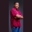Related Tags

swift
communitycreator

# How to create a hexadecimal value in Swift with 0xTheodore Kelechukwu Onyejiaku

### Overview

A hexadecimal value is a value in the number system that is base 16. By default, all values are in the decimal base, that is base 10. In Swift, we create a hexadecimal value by placing 0x before the digits, we want.

### Syntax

0x(hexadecimalDigit)
Syntax to create haxadecimal value with 0x

### Parameters

• 0x: This is the keyword for creating a hexadecimal value in Swift.
• hexadecimalDigit: This is the hexadecimal digit we want to use. It can be any of the hexadecimal digits like A , C, etc.

### Return value

The value returned by this keyword is a hexadecimal value.

NOTE: When we print the created hexadecimal value to the console, we will get its equivalent decimal value.

### Example

// create variables with hexadecimal values
let hex1 = 0x1;
let hex2 = 0xc3;
let hex3 = 0x14;
let hex4 = 0xAC2;

// print the decimal equivalents
print(hex1)
print(hex2)
print(hex3)
print(hex4)

### Explanation

• Lines 2–5: We create some variables and initialize them with some hexadecimal values.
• Lines 8–11: We print the values in their decimal equivalents to the console.

RELATED TAGS

swift
communitycreator

CONTRIBUTORTheodore Kelechukwu Onyejiaku
RELATED COURSES

View all Courses

Keep Exploring

Learn in-demand tech skills in half the time